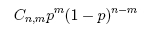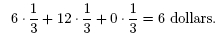# Probability Review

The following concepts should help you solve the problems in each activity.

#### Independent Events

Suppose you choose a random number from 1 to 3. Then the probability of choosing the number 1 is 1/3. If you were to do this twice in a row, then the probability of choosing 1 both times would be 1/3 times 1/3, that is, 1/9. In general, if you have two unrelated (also called independent) events, then the probability of them both occurring is the product of the probabilities of each of the individual events.

#### Probabilities Sum to One

Each time we run a trial, with probability one we get some outcome. Therefore, the sum of the probabilities of all possible outcomes must be one. This allows you to calculate the probability of an event by finding the probability that the event does not occur and subtracting this probability from one. In many situations this is much easier than calculating the probability directly. For example, in the previous situation, if you want to find the probability of choosing any pair except for two 1's, then, since you know that the probability of actually getting two 1's is 1/9, the probability of not getting the pair of 1's must be 1 - [probability of getting the pair of 1's] = 1 - 1/9 = 8/9.

#### Factorials

5 people can stand in a line in 5! = 5 · 4 · 3 · 2 · 1 = 120 different ways (the first person in line can be any of the five people, the second person any of the remaining four, the third person any of the remaining three, and so on...). The symbol 5! is read as "five factorial". For n people the answer is n! = n · (n - 1) · · · 2 · 1 ways.

#### Permutations

A club with 23 members can elect a president, vice-president, secretary, and treasurer in 23 · 22 · 21 · 20 ways. In general, for n people and m offices there are nPm = n · (n-1) · (n-2) · · · (n-m+1) = n!/(n-m)! different ways.

#### Combinations

From a group of size 23 you can pick a committee of size four in (23 · 22 · 21 · 20)/4! ways (if we make a list of the permutations in the previous problem, each committee appears 4! times. When choosing the committee, none of the 4 positions are distinguished, so the order in which we choose the four committee member does not matter). Picking m things out of n can be done in Cn,m = n!/[m!(n-m)!] different ways. This is often read as "n choose m". The numbers Cn,m are also called binomial coefficients.

#### Binomial Distribution

The binomial distribution tells us that the probability of getting m successes in n trials when the success probability is p isThe first factor gives the number of ways to pick the m trials on which success happens. The second gives the probability of any particular outcome with m successes and n-m failures.

#### Expected value

In a situation where you have a set of outcomes each with a given probability of occurring and each with some numerical "payoff" value, you might be interested in what average payoff you will earn. This average payoff, also called the expected value, is calculated by taking the sum over all possible outcomes of the probability of each outcome times the payoff for that outcome. For example, suppose that you are to choose one door among three possible choices. Behind one door there are 6 dollars, behind another one there are 12 dollars and behind the third door there is nothing, but you don't know what is behind each door. Then the expected payoff you will earn is the sum over all possible doors of probability of choosing each door (in this case 1/3 for each door) times the payoff for that door: